# Mathematics facts for kids

Kids Encyclopedia Facts
(Redirected from Math)

Mathematics is the study of numbers, shapes and patterns. The word is sometimes shortened to maths (in England, Australia, and New Zealand) or math (in the United States and Canada). Mathematicians are people who do mathematics. Mathematics is useful for solving problems that occur in the real world, so many people besides mathematicians study and use mathematics. Today, some mathematics is needed in many jobs. Business, science, engineering, and construction need some knowledge of mathematics.

Mathematicians solve problems by using logic. Mathematicians often use deduction. Deduction is a special way of thinking to discover and prove new truths using old truths. To a mathematician, the reason something is true is just as important as the fact that it is true. Using deduction is what makes mathematical thinking different from other kinds of thinking.

Mathematics includes the study of:

• Numbers (example 3+6=9)
• Structure: how things are organized.
• Place: where things are and their arrangement.
• Change: how things become different over time.

Mathematics uses logic, paper, and calculator. These things are used to create general rules, which are an important part of mathematics. These rules leave out information that is not important so that a single rule can cover many situations. By finding general rules, mathematics solves many problems at the same time as these rules can be used on other problems.

A proof gives a reason why a rule in mathematics is correct. This is done by using certain other rules that everyone agrees are correct, which are called axioms. A rule that has a proof is sometimes called a theorem. Experts in mathematics perform research to create new theorems. Sometimes experts find an idea that they think is a theorem but can not find a proof for it. That idea is called a conjecture until they find a proof.

Sometimes, mathematics finds and studies rules or ideas in the real world that we don't understand yet. Often in mathematics, ideas and rules are chosen because they are considered simple or neat. On the other hand, sometimes these ideas and rules are found in the real world after they are studied in mathematics; this has happened many times in the past. In general, studying the rules and ideas of mathematics can help us understand the world better.

### Number

Mathematics includes the study of number, or quantity.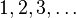$1, 2, 3, \ldots$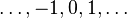$\ldots, -1, 0, 1, \ldots$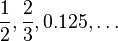$\frac{1}{2}, \frac{2}{3}, 0.125,\ldots$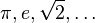$\pi, e, \sqrt{2},\ldots$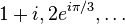$1+i, 2e^{i\pi/3},\ldots$ Natural numbers Integers Rational numbers Real numbers Complex numbers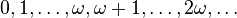$0, 1, \ldots, \omega, \omega + 1, \ldots, 2\omega, \ldots$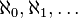$\aleph_0, \aleph_1, \ldots$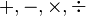$+,-,\times,\div$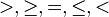$>,\ge, =, \le, <$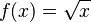$f(x) = \sqrt x$ Ordinal numbers Cardinal numbers Arithmetic operations Arithmetic relations Functions

### Structure

Some areas of mathematics study the structure that an object has.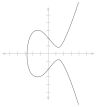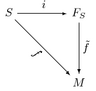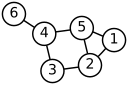Number theory Abstract algebra Linear algebra Order theory Graph theory

### Shape

Some areas of mathematics study the shapes of things.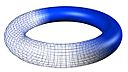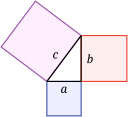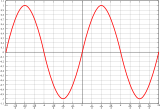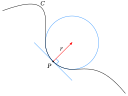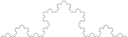Topology Geometry Trigonometry Differential geometry Fractal geometry

### Change

Some areas of mathematics study the way things change.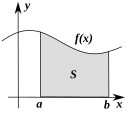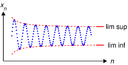Calculus Vector calculus Analysis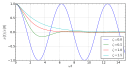Differential equations Dynamical systems Chaos theory

### Applied mathematics

Applied mathematics uses mathematics to solve problems of other areas such as engineering, physics, and computing.
Numerical analysis – Optimization – Probability theoryStatistics – Mathematical finance – Game theoryMathematical physicsFluid dynamics - computational algorithms

## Famous theorems

These theorems have interested mathematicians and people who are not mathematicians.

Pythagorean theoremFermat's last theoremGoldbach's conjectureTwin Prime ConjectureGödel's incompleteness theoremsPoincaré conjectureCantor's diagonal argumentFour color theorem – Zorn's lemma – Euler's IdentityChurch-Turing thesis

These are theorems and conjectures that have greatly changed mathematics.

Riemann hypothesisContinuum hypothesisP Versus NPPythagorean theoremCentral limit theoremFundamental theorem of calculusFundamental theorem of algebraFundamental theorem of arithmetic – Fundamental theorem of projective geometry – classification theorems of surfaces – Gauss-Bonnet theoremFermat's last theorem

## Foundations and methods

Progress in understanding the nature of mathematics also influences the way mathematicians study their subject.

Philosophy of mathematics – Mathematical intuitionism – Mathematical constructivism – Foundations of mathematics – Set theory – Symbolic logic – Model theory – Category theoryLogic – Reverse Mathematics – Table of mathematical symbols

### History and the world of mathematicians

Mathematics in history, and the history of mathematics.

History of mathematics – Timeline of mathematics – MathematiciansFields medal – Abel Prize – Millennium Prize Problems (Clay Math Prize) – International Mathematical Union – Mathematics competitions – Lateral thinking – Maths and gender

## Name

The word "mathematics" comes from the Greek word "μάθημα" (máthema). The Greek word "μάθημα" means "science, knowledge, or learning".

Often, the word "mathematics" is made shorter into maths (in British English) or math (in American English). The short words maths or math are often used for arithmetic, geometry or simple algebra by students and their schools.

## Awards in mathematics

There is no Nobel prize in mathematics. Mathematicians can receive the Abel prize and the Fields Medal for important works.

The Clay Mathematics Institute has said it will give one million dollars to anyone who solves one of the Millennium Prize Problems

## Mathematical tools

There are many tools that are used to do mathematics or to find answers to mathematics problems.

Older tools